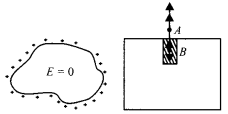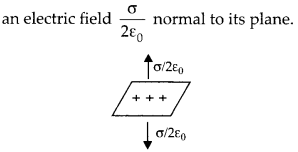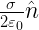Enlightened

# Question 29: NCERT Class 12th Physics: Chapter 1 – Electric Charges And Fields

• 0

Question 29: NCERT Class 12th Physics: Chapter 1 – Electric Charges And Fields

A hollow charged conductor has a tiny hole cut into its surface. Show that the electric field in the hole is.whereis the unit vector in the outward normal direction, and o is the surface charge density near the hole.

Share

1. Solution:
Inside a charged conductor, the electric field is zero.But a uniformly charged flat surface provideIf we consider a small flat part on the surface of charged conductor, it certainly provides anNow if a hole is made in charged conductor, the fifeld due to small flat part is absent but the field due to rest of charged conductor is present i.e., equal toNCERT 12th Physics Chapter 1, Class 12 Physics Chapter 1 solutions

Check the complete chapter with solutions.

NCERT Solutions for 12th Class Physics: Chapter 1-Electric Charges And Fields

• 0